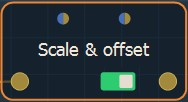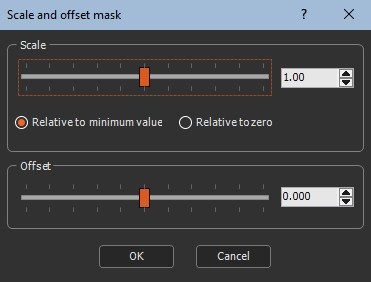### Adding a Scale & offset node

This node sets the scale and offset of the mask.

To add a Scale & offset node, right-click in the Graph Editor and select Create Node  > Mask Transformation  > Scale & offset.Double click on the node to open its parameters:### Editing the scale and offset

• Use the Scale slider to change the scale of the mask size.
• Set to what the scale is relative:
• Relative to minimum height: Minimum value of the mask heights does not change. If the mask heights are between -20 and 80 (range 100), and with a factor of 2, the heights are between -20 and 180 (range 200).
• Relatively to zero: Zero heights do not change. If the mask heights are between -20 and 80 (range 100), and with a factor of 2, the heights are between -40 and 160 (range 200).
• Offset: Sets the positive or negative offset to apply.

### Parameters

Parameter Use
Scale Scale factor to be applied.
Relatively to minimum height Minimum value of the mask heights does not change. If the mask heights are between -20 and 80 (range 100), and with a factor of 2, the heights are between -20 and 180 (range 200).
Relatively to zero Zero heights do not change. If the mask heights are between -20 and 80 (range 100), and with a factor of 2, the heights are between -40 and 160 (range 200).
Offset The offset (positive or negative) to apply.